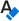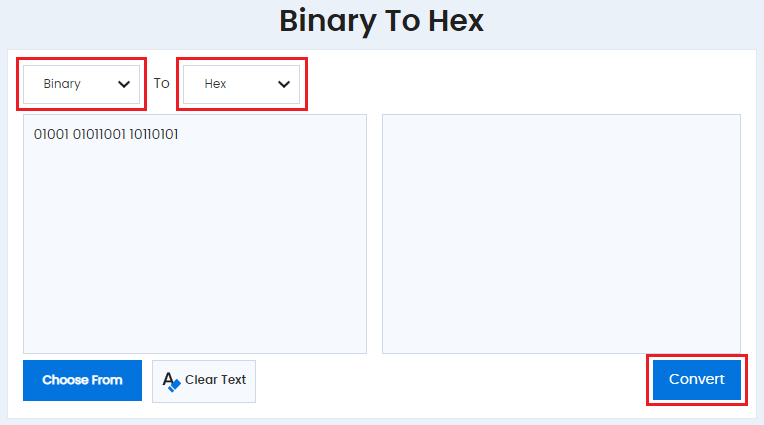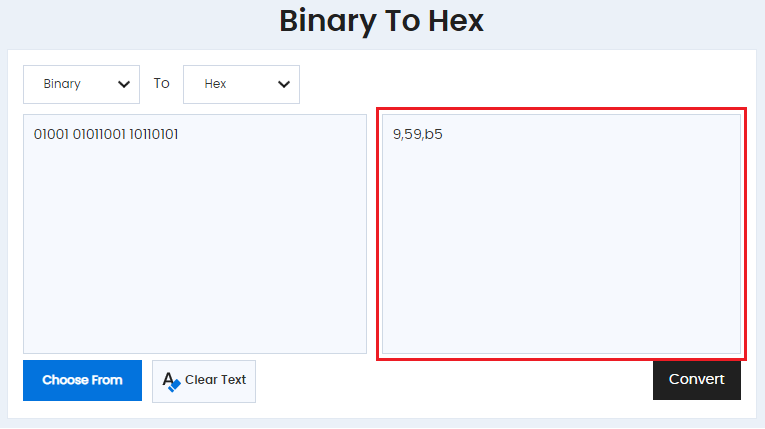# Binary To Hex

How to convert Binary to Hexadecimal? Instantly convert binary characters with this hex conversion. Binary to hex translator by just giving your binary digits and One click button to get your results in Hexadecimal.

ToThe programming of Binary digits are formed in such a sequence that the characters are readable in the Hex values. The binary data associated with the data is essential when encoding and it is represented in a range from 00 to FF. The numbers 0–9 represent numbers up to nine whereas and a-f is represented by the numeric 10–15.

### How to convert binary to hexadecimal by hand ?

A grouping method is used in changing arithmetic number from binary to hex. The binary number is then segregated from the hex converter values into groups of four digits starting from the right. The formed groups are then converted to hexadecimal digits. The grouping is then removed once the conversion has been made and the results are in Hex digits.

Number Conversions & their Arithmetic

With binary digits to Hex conversion tool, we have eliminated the hassle of counting and converting the lengthy 0 and 1 calculations to convert them into Hexadecimal. All you have to do is use the binary to Hex online converter offered for free to represent text or instructions to the computer easily. You also have an option to swap the Hex to Binary or Binary to Hex, just the way you want your values to be converted into. There might include numerous amounts of combinations of 0 and 1 which can easily confuse anyone.

• Get started by visiting https://www.duplichecker.com/binary-to-hex.php
• Add the combination of 0 and 1 that you want to convert into hex digits after selecting the Binary unit in the left box. Then all you have to do is select the unit in the box next to it on the right that has Hex unit option and click “Convert.”• It is as easy as it can get. The result will then appear in the right box, and you can also swap the units to find results at any time.Hexadecimal often shortened as hex is a system based on the base 16 that is used to simplify how binary is represented (Binary representation). The hex to binary converter, numeral system is a 16 symbols numeral system developed so that an 8-bit binary number can be written.

It can be inscribed using only two diverse hex digits - one hex digit illustrating each nibble or either in 4-bits. The system is more successful as it is relatively much easier to write numbers as hex than to write them in the structure of the binary number representation.

The standard numeral system is called decimal (base 10) which can be of the following 0,1,2,3,4,5,6,7,8,9. Hexadecimal converter uses the decimal numbers and depicts six extra symbols A B C D E F. Letters which are taken from the English alphabets used as the numerical symbols lack in representing the values which are greater than ten. For instance, Hexadecimal “A” represents decimal 10, and hexadecimal “F” portrays the decimal 15.

Pro tip [1# Hex to text 2# Hex to Decimal 3# Hex to Binary]

Humans mostly use the decimal system as machines generally use a binary representation language system. This could be a coincidence that the word humans have ten fingers (ten digits). However, computers are only dependent on using on and off, called a binary digit.

### Most Significant Bit

On the other hand, a binary system has a string of zeros and ones that are encoded into the computers to receive and provide a command, 11011011, for example. For a more precise understanding, professionals working with computers tend to group bits.

In the early 1960s, they would form group 3 bits for the time being used. Three bits, each with a function of commanding on or off in the machine, can represent the eight numbers from 0 to 7.

The formation is called octal that forms the 3 bit digits as 000 = 0; 001 = 1; 010 = 2; 011 = 3; 100 = 4; 101 = 5; 110 = 6 and 111 = 7.

### Furthermore, Convert from:

#### Table of Binary, Hexadecimal and ASCII values,

00000000 00 NUL
00000001 01 SOH
00000010 02 STX
00000011 03 ETX
00000100 04 EOT
00000101 05 ENQ
00000110 06 ACK
00000111 07 BEL
00001000 08 BS
00001001 09 HT
00001010 0A LF
00001011 0B VT
00001100 0C FF
00001101 0D CR
00001110 0E SO
00001111 0F SI
00010000 10 DLE
00010001 11 DC1
00010010 12 DC2
00010011 13 DC3
00010100 14 DC4
00010101 15 NAK
00010110 16 SYN
00010111 17 ETB
00011000 18 CAN
00011001 19 EM
00011010 1A SUB
00011011 1B ESC
00011100 1C FS
00011101 1D GS
00011110 1E RS
00011111 1F US
00100000 20 Space
00100001 21 !
00100010 22 "
00100011 23 #
00100100 24 \$
00100101 25 %
00100110 26 &
00100111 27 '
00101000 28 (
00101001 29 )
00101010 2A *
00101011 2B +
00101101 2D -
00101110 2E .
00101111 2F /
00110000 30 0
00110001 31 1
00110010 32 2
00110011 33 3
00110100 34 4
00110101 35 5
00110110 36 6
00110111 37 7
00111000 38 8
00111001 39 9
00111010 3A :
00111011 3B ;
00111100 3C <
00111101 3D =
00111110 3E >
00111111 3F ?
01000000 40 @
01000001 41 A
01000010 42 B
01000011 43 C
01000100 44 D
01000101 45 E
01000110 46 F
01000111 47 G
01001000 48 H
01001001 49 I
01001010 4A J
01001011 4B K
01001100 4C L
01001101 4D M
01001110 4E N
01001111 4F O
01010000 50 P
01010001 51 Q
01010010 52 R
01010011 53 S
01010100 54 T
01010101 55 U
01010110 56 V
01010111 57 W
01011000 58 X
01011001 59 Y
01011010 5A Z
01011011 5B [
01011100 5C \
01011101 5D ]
01011110 5E ^
01011111 5F _
01100000 60 `
01100001 61 a
01100010 62 b
01100011 63 c
01100100 64 d
01100101 65 e
01100110 66 f
01100111 67 g
01101000 68 h
01101001 69 i
01101010 6A j
01101011 6B k
01101100 6C l
01101101 6D m
01101110 6E n
01101111 6F o
01110000 70 p
01110001 71 q
01110010 72 r
01110011 73 s
01110100 74 t
01110101 75 u
01110110 76 v
01110111 77 w
01111000 78 x
01111001 79 y
01111010 7A z
01111011 7B {
01111100 7C |
01111101 7D }
01111110 7E ~
01111111 7F DEL